## Here I will update new post very soon

Hi, its long time. Now I start again to post new articles. Cant wait….

First, I push some new tips related R-studio etc.

Kind Regards,
Marko

## Finding Centroid point from shape file and labelling map

Finding Centroid point from shape file and labelling map

# Finding Centroid point from shape file and map data labelling

In this test I try to find centroid point in shp file. In additionally I will show how to use this centroid point information when to labelling area name into map.
R Library PBSmapping offers wide range of functions to do that job.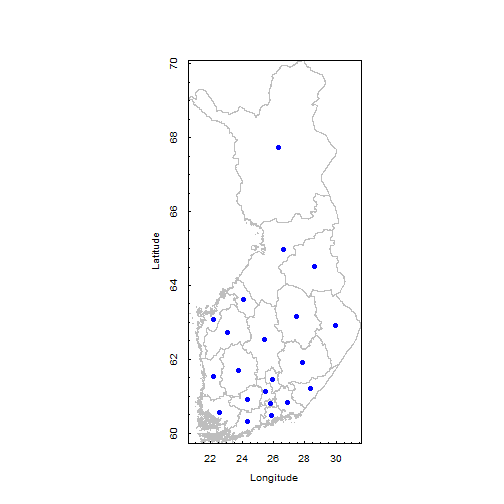## Needed Library

require(rgdal)

## Loading required package: rgdal

## Loading required package: sp

## Warning: package 'sp' was built under R version 2.15.1

## Geospatial Data Abstraction Library extensions to R successfully loaded
## Loaded GDAL runtime: GDAL 1.9.0, released 2011/12/29 Path to GDAL shared
## files: C:/Program Files/R/R-2.14.2/library/rgdal/gdal Loaded PROJ.4
## runtime: Rel. 4.7.1, 23 September 2009, [PJ_VERSION: 470] Path to PROJ.4
## shared files: C:/Program Files/R/R-2.14.2/library/rgdal/proj

require(PBSmapping)

## Loading required package: PBSmapping

## ----------------------------------------------------------- PBS Mapping
##
## PBS Mapping comes with ABSOLUTELY NO WARRANTY; for details see the file
## COPYING. This is free software, and you are welcome to redistribute it
## under certain conditions, as outlined in the above file.
##
## A complete user guide 'PBSmapping-UG.pdf' is located at C:/Program
## Files/R/R-2.14.2/library/PBSmapping/doc/PBSmapping-UG.pdf
##
## To see demos, type '.PBSfigs()'.
##
## Packaged on 2012-03-01 Pacific Biological Station, Nanaimo
## -----------------------------------------------------------

require(maptools)

## Loading required package: maptools

## Loading required package: foreign

## Loading required package: lattice

## Checking rgeos availability: TRUE


## Importing Shapefile

FIN_adm2.pb <- importShapefile("FIN_adm2")


ImportShapefile reads the .prj file if it exists, but it
does not adopt the proj4 format

proj.abbr <- attr(FIN_adm2.pb, "projection")
# abbreviated projection info


print(proj.abbr)

## Generate map using PBSmapping plotting functions

plotPolys(FIN_adm2.pb, projection = proj.abbr,
border = "gray", xlab = "Longitude", ylab = "Latitude")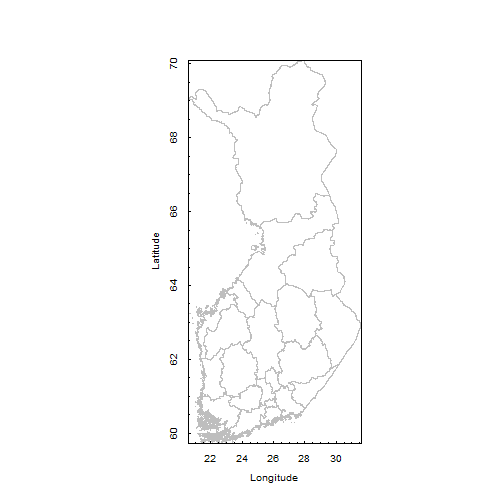## Calculate the centroids of adm2 area

FIN.centroids2 <- calcCentroid(FIN_adm2.pb, rollup = 1)


Check results

str(FIN.centroids2)

## Classes 'PolyData' and 'data.frame': 21 obs. of  3 variables:
##  $PID: int 1 2 3 4 5 6 7 8 9 10 ... ##$ X  : num  29.9 27.5 26 27.9 26.3 ...
##  $Y : num 62.9 63.2 61.4 61.9 67.7 ... ## - attr(*, "projection")= chr "LL"  ## Plot the centroids against the source polygons In this purpose we use the PBSMapping package graphics routines. If you want you can plot these centroid point in to the map plotPolys(FIN_adm2.pb, projection = proj.abbr, border = "gray", xlab = "Longitude", ylab = "Latitude") addPoints(FIN.centroids2, col = "blue", pch = 19)check the variables from imported shape file str(FIN_adm2.pb)  ## Classes 'PolySet' and 'data.frame': 136271 obs. of 5 variables: ##$ PID: int  1 1 1 1 1 1 1 1 1 1 ...
##  $SID: int 1 1 1 1 1 1 1 1 1 1 ... ##$ POS: int  1 2 3 4 5 6 7 8 9 10 ...
##  $X : num 28.5 28.5 28.5 28.5 28.6 ... ##$ Y  : num  63.9 63.9 63.9 63.9 63.9 ...
##  - attr(*, "PolyData")=Classes 'PolyData' and 'data.frame':  21 obs. of  12 variables:
##   ..$PID : int 1 2 3 4 5 6 7 8 9 10 ... ## ..$ ID_0     : int  78 78 78 78 78 78 78 78 78 78 ...
##   ..$ISO : Factor w/ 1 level "FIN": 1 1 1 1 1 1 1 1 1 1 ... ## ..$ NAME_0   : Factor w/ 1 level "Finland": 1 1 1 1 1 1 1 1 1 1 ...
##   ..$ID_1 : int 1 1 1 1 2 3 3 4 4 4 ... ## ..$ NAME_1   : Factor w/ 5 levels "Eastern Finland",..: 1 1 1 1 2 3 3 4 4 4 ...
##   ..$ID_2 : int 1 2 3 4 5 6 7 8 9 10 ... ## ..$ NAME_2   : Factor w/ 19 levels "Central Finland",..: 8 9 13 17 7 5 10 3 6 13 ...
##   ..$NL_NAME_2: Factor w/ 0 levels: NA NA NA NA NA NA NA NA NA NA ... ## ..$ VARNAME_2: Factor w/ 19 levels "Etelä-Karjala|Södra Karelen",..: 12 14 15 3 10 5 13 4 9 15 ...
##   ..$TYPE_2 : Factor w/ 1 level "Maakunta|landskap": 1 1 1 1 1 1 1 1 1 1 ... ## ..$ ENGTYPE_2: Factor w/ 1 level "Region": 1 1 1 1 1 1 1 1 1 1 ...
##  - attr(*, "parent.child")= num  1 1 1 1 0 1 0 0 0 0 ...
##  - attr(*, "shpType")= int 5
##  - attr(*, "prj")= __truncated__
##  - attr(*, "projection")= chr "LL"


To read dbf file we need foreign library

library(foreign)


reading shape file data from dbf file (ESRI files include normally: shp, dbf, prj, csv and shx data files)

labelData <- read.dbf("FIN_adm2.dbf", as.is = TRUE)


Data checking

head(labelData)

##   ID_0 ISO  NAME_0 ID_1          NAME_1 ID_2            NAME_2 NL_NAME_2
## 1   78 FIN Finland    1 Eastern Finland    1     North Karelia      <NA>
## 2   78 FIN Finland    1 Eastern Finland    2     North Savonia      <NA>
## 3   78 FIN Finland    1 Eastern Finland    3 Päijänne Tavastia      <NA>
## 4   78 FIN Finland    1 Eastern Finland    4  Southern Savonia      <NA>
## 5   78 FIN Finland    2         Lapland    5           Lapland      <NA>
## 6   78 FIN Finland    3            Oulu    6            Kainuu      <NA>
##                         VARNAME_2            TYPE_2 ENGTYPE_2
## 1   Pohjois-Karjala|Norra Karelen Maakunta|landskap    Region
## 2      Pohjois-Savo|Norra Savolax Maakunta|landskap    Region
## 3 Päijät-Häme|Päijänne Tavastland Maakunta|landskap    Region
## 4        Etelä-Savo|Södra Savolax Maakunta|landskap    Region
## 5                  Lappi|Lappland Maakunta|landskap    Region
## 6               Kainuu|Kajanaland Maakunta|landskap    Region


merging shape files area name and centroid points (x and y coordinates) labelData as data frame

labelData <- as.data.frame(labelData)


merging labelData and centroid data table fields

label <- data.frame(labelData$ID_2, FIN.centroids2$X,
FIN.centroids2$Y, labelData$NAME_2)


Event data function needs right column names

names(label) <- c("EID", "X", "Y", "label")


Note: Above routine used in eventdata function

#######names(label)<- c(“PID”, “X”, “Y”, “label”) –> USED IN POLYDATA FUNCTION

do the right data format into labelling

data <- as.EventData(label, projection = NULL,
zone = NULL)


## Labelling into map

#col = 1 –> font color 1=black, 2=….
#font = 3 –> font size
#cex = 0.5 –>expansion factor

plotPolys(FIN_adm2.pb, projection = proj.abbr,
border = "gray", xlab = "Longitude", ylab = "Latitude")

col = 1, font = 3, cex = 0.5)

## Warning: The data's 'projection' attribute (NULL) differs from the
## projection of the plot region (LL).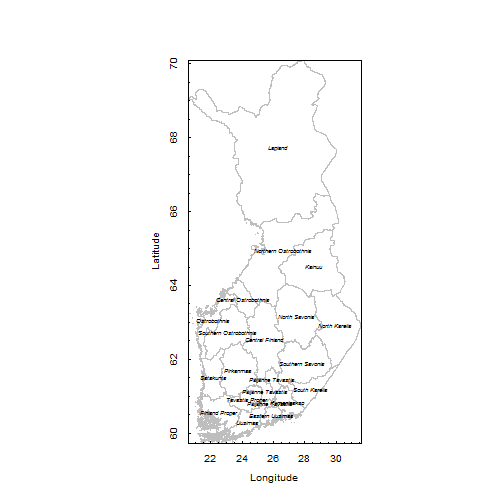Have fun with mapping and RStudio

Marko

ps. This post is produced by using RStudio and knitr. Thanks to Yihui Xie for amazing work with this issue.

# Data Envelopment Analysis

Within couple of days I have been testing DEA modelling (Data Envelopment Analysis) with different R-package. Finally,…. I have found such a comprehensive way to calculate Malmquist indices.

My primary purpose is to show how to use nonparametric methods for measuring efficiency and productivity by using R-programs nonparaeff -package.At this same time I will show you how to present Malmquist indices within googleVis -world map and finally I will introduse how to make forecast chart for productivity (Chart 1), effectiveness and technical effectiveness indices. In this work I used R-program forecast -library.

Used R-library documentation:
nonparaeff
forecast

First of all I would like to thank Author Dong-hyun Oh for nice work with this nonparaeff-package.

In this working paper I will use example of faremalm2. Like nonpraeff -package documentation we calculate Malmquist productivity growth index of OECD countries
(productivity, technical efficiency and efficiency). As data source we have used Penn World Table (like original sources) with following version:
OECD Timeseries 1980-1990  version pwt5.6
OECD Timeseries 1990-2009  version pwt7.0
In pwt7.0 I cannot find capital stock data, so I downloaded it from here (http://www.ifw-kiel.de/forschung/datenbanken/netcap)

Productivity calculation 1980-1990 As output variables is used  Total GDP of a country. This variables  is calculated using GDP per capita (rgdpl)
and amount of total population (pop). As input variables is used Total labor force (gdp/rgdpwok) and Total capital stock  (kapw * labor)

In second productivity computing calculation 1990-2009 I only use one input and one output variables (labor and gdp). This is because there is no
capital stock data available in pwt7.0 (please correct this If I missed something). I will re-calculate this period asap when I get capital data from all countries.

In third productivity computing 1992-2002 I use pwt7.0 and get capital stock data from different OECD datasource
(http://www.ifw-kiel.de/forschung/datenbanken/netcap). As input I used labor and capital variables and as output variables gdp.

When use faremalm2 function you will get the following outputs:
pc.c –> Productivity growth for each country
pc.y  –> A trend of productivity growth of SELECTED countries (in this work we use OECD)

tc.c –> Technical efficiency  for each country
tc.y –> A trend of technical efficiency growth of SELECTED countries

ec.c –> Efficiency change for each country
ec.y –> A trend of efficiency change of SELECTED countries

## Useage of faremalm2

faremalm2(dat = NULL, noutput = 1, id = “id”, year = “year”)
dat –> The format of this data frame is data.frame(id, year, outputs, inputs).
noutput –> number of outputs
id –> column name for DMU:s
year –> column name for the time index

## Malmquist productivity growth index of OECD countries #First install data from OECD install.packages("pwt")  # (installing a package can take a couple of minutes) 

#Setting the library into use library(pwt) ## Use Penn World Table library(nonparaeff) # DEA modelling 

#my.dat <- pwt5.6  #used in 1980-1990 #my.dat <- pwt6.3  # my.dat <- pwt7.0  #used in 1990-2009 and 1992-2002 #head(my.dat) summary(my.dat) #my.dat$country  #capital stock data missing so we get it from there # http://www.ifw-kiel.de/forschung/datenbanken/netcap #picking up OECD countries my.oecd.ctry <- c("AUS", "AUT", "BEL", "CAN", "CHE", "DNK", "ESP", "FIN", "FRA", "GBR", "GER", "GRC", "IRL", "ISL", "ITA", "JPN", "KOR", "LUX", "MEX", "NLD", "NOR", "NZL", "PRT", "SWE", "TUR", "USA", "DEU")  #NOTE WPCODE USED IN OLDER DATASET #adding country code #my.dat <- my.dat[my.dat$wbcode %in% my.oecd.ctry,]  #use this with pwt5.6 my.dat <- my.dat[my.dat$isocode %in% my.oecd.ctry,] #use this with pwt7.0 summary(my.dat) #selecting appropriate years #my.dat <- my.dat[my.dat$year %in% 1980:1990,] #my.dat <- my.dat[my.dat$year %in% 1950:1992,] my.dat <- my.dat[my.dat$year %in% 1990:2009,] #my.dat <- my.dat[my.dat$year %in% 1992:2002,] summary(my.dat) my.dat Note! I encounter problem (NA or other reason) thats why I do this out of box. my.dat is transformed into data.frame and after that modified with Excel. This is only used in timeseries 1992-2002 productivity calculation. #uploading my.dat #my.dat_df <- as.data.frame(my.dat) #out #write.table(my.dat_df, file = "c:/data/my.dat_df.txt", sep = "\t", col.names = NA, qmethod = "double") Capital stock data inserted in this phase. source: http://www.ifw-kiel.de/forschung/datenbanken/netcap #...and in both source my.dat <- read.table(".../mydat1990_2009.csv", header=TRUE, sep=";", na.strings="NA", dec=",", strip.white=TRUE) my.dat <- read.table("http://ekqvist.goeuropeinfo.com/rbloggerqvist/ data/oecd/mydat1990_2009.csv", header=TRUE, sep=";", na.strings="NA", dec=",", strip.white=TRUE) #INSERTING some variables to my.dat (ie. INPUT and OUTPUT -variables calculation) my.dat$rgdpl <- as.numeric(my.dat$rgdpl) ## GDP per capita my.dat$pop <- as.numeric(my.dat$pop) ## total population (1000) my.dat$rgdpwok <- as.numeric(my.dat$rgdpwok) ## GDP per labor my.dat$gdp <- my.dat$rgdpl * my.dat$pop ## Total GDP of a country my.dat$labor <- with(my.dat, gdp/rgdpwok) ## Total labor force my.dat$capital <- with(my.dat, kapw * labor) ## Total capital stock           MISSING pwt7 note: this is used in pwt5.6
#my.dat$capital <- as.numeric(my.dat$capital)
## Total capital stock  ADDED FROM DIFF. SOURCE now used in time
series 1993-2002
my.dat$kapw <- as.numeric(my.dat$kapw) ## Capital stock per labor         MISSING pwt7 note: this is used in pwt5.6
#my.dat$kapw <- with(my.dat, my.dat$capital/my.dat$labor) ## Capital stock per labor now used in time series 1993-2002 #variable used in 1990-2009 Malmquist productivity calculation #1980-1990 #1990-2009 #1992-2002 #these variables used in timeseries 1980-1990 and 1992-2002 #oecd <- my.dat[, c("country", "wbcode", "year", "gdp", "labor", "capital")] #used in time series 1980-1990 oecd <- my.dat[, c("country", "isocode", "year", "gdp", "labor")] # 1990-2009 #oecd <- my.dat[, c("country", "isocode", "year", "gdp", "labor", "capital")] #used in time series 1992-2002 summary(oecd) head(oecd)  #removing NA-rows oecd <- oecd[!is.na(oecd$capital),] summary(oecd)

#Now calcuatel productivity (pc), effiency (ec) and technical efficiency (tc) #huom. tämä on tehty eri aikasarjoille ja hyvä niin koska mahdollistaa eri aikajaksojen tarkastelun library(nonparaeff) # DEA modelling re.oecd <- faremalm2(dat = oecd, noutput = 1, id = "isocode", year = "year") #re.oecd <- faremalm2(dat = oecd, noutput = 1, id = "wbcode", year = "year")

summary(re.oecd)

###################################################### #ISOCODE USED IN #1990-2009 and 1992-2002 #note:

## productivity growth for each country pc.c <- tapply(re.oecd$pc, re.oecd$isocode, geometric.mean) #pc.c <- tapply(re.oecd$pc, re.oecd$wbcode, geometric.mean) ## a trend of productivity growth of SELECTED countries pc.y <- tapply(re.oecd$pc, re.oecd$year, geometric.mean)

## technical efficiency  for each country
tc.c <- tapply(re.oecd$tc, re.oecd$isocode, geometric.mean)
#tc.c <- tapply(re.oecd$tc, re.oecd$wbcode, geometric.mean)

## a trend of technical efficiency growth of SELECTED  countries
tc.y <- tapply(re.oecd$tc, re.oecd$year, geometric.mean)

## efficiency change for each country
ec.c <- tapply(re.oecd$ec, re.oecd$isocode, geometric.mean)
#ec.c <- tapply(re.oecd$ec, re.oecd$wbcode, geometric.mean)
## a trend of efficiency change of SELECTED  countries
ec.y <- tapply(re.oecd$ec, re.oecd$year, geometric.mean)
######################################################
pc.c

#1980-1990, 1990-2009 #country -variable(we use it in google vis plotting) ## productivity growth for each country pc.c <- tapply(re.oecd$pc, re.oecd$country, geometric.mean)

## technical efficiency  for each country
tc.c <- tapply(re.oecd$tc, re.oecd$country, geometric.mean)

## efficiency change for each country
ec.c <- tapply(re.oecd$ec, re.oecd$country, geometric.mean)

 #missing time series 1992-2002 TURKEY, KOREA, LUXEMBOURG AND MEKSIKO capital stock data #VUOSITTAINEN startyear-endyear KESKIARVO TEHOKKUUSKERTOIMESSA (MALMQUIST - FARREL) #ec: efficiency change  #TEHOKKUUSMUUTOS ec.c_df <- as.data.frame(ec.c) summary(ec.c_df) ec.c_df #tc: technical change  #TEKNISEN TEHOKKUUDEN MUUTOS tc.c_df <- as.data.frame(tc.c)

#pc: productivity change  #TUOTTAVUUDEN MUUTOS
pc.c_df <- as.data.frame(pc.c)

#LITTLE TRICK #ec: efficiency change  #TEHOKKUUSMUUTOS write.table(ec.c_df, file = "c:/data/ec.c_df.txt", sep = "\t", col.names = NA, qmethod = "double") ec.c_df2  <- read.table("c:/data/ec.c_df.txt",  header=TRUE, sep="\t", na.strings="NA", dec=".", strip.white=TRUE) names(ec.c_df2)<- c("Country", "Effiency")

 #tc: technical change  #TEKNISEN TEHOKKUUDEN MUUTOS write.table(tc.c_df, file = "c:/data/tc.c_df.txt", sep = "\t", col.names = NA, qmethod = "double") tc.c_df2  <- read.table("c:/data/tc.c_df.txt",  header=TRUE, sep="\t", na.strings="NA", dec=".", strip.white=TRUE) names(tc.c_df2)<- c("Country", "Effiency")

#pc: productivity change  #TUOTTAVUUDEN MUUTOS
write.table(pc.c_df, file = “c:/data/pc.c_df.txt”, sep = “\t”, col.names = NA, qmethod = “double”)
names(pc.c_df2)<- c(“Country”, “Effiency”)

#REMOVING NA-VALUES ec.c_df2 <- ec.c_df2[!is.na(ec.c_df2$Effiency),] tc.c_df2 <- tc.c_df2[!is.na(tc.c_df2$Effiency),] pc.c_df2 <- pc.c_df2[!is.na(pc.c_df2$Effiency),] summary(ec.c_df2) #just cecking country names ec.c_df2$Country

#replace some country name with new one…
ec.c_df3 <- gsub(“United States of America”, “United States”, ec.c_df2$Country) ec.c_df3 <- gsub(“Germany, West”, “Germany”, ec.c_df3) ec.c_df3 <- gsub(“Korea, Republic”, “South Korea”, ec.c_df3) tc.c_df3 <- gsub(“United States of America”, “United States”, tc.c_df2$Country)
tc.c_df3 <- gsub(“Germany, West”, “Germany”, tc.c_df3)
tc.c_df3 <- gsub(“Korea, Republic”, “South Korea”, tc.c_df3)

pc.c_df3 <- gsub(“United States of America”, “United States”, pc.c_df2$Country) pc.c_df3 <- gsub(“Germany, West”, “Germany”, pc.c_df3) pc.c_df3 <- gsub(“Korea, Republic”, “South Korea”, pc.c_df3) #as a dataframe MUUNNETAAN DATA FRAMEKSI ec.c_df3 <- as.data.frame(ec.c_df3) tc.c_df3 <- as.data.frame(tc.c_df3) pc.c_df3 <- as.data.frame(pc.c_df3) #check total number of rows KATSOTAAN RIVINUMEROINNIT MERGELLE summary(ec.c_df2) nrow(ec.c_df2) nrow(ec.c_df3) summary(tc.c_df2) nrow(tc.c_df2) nrow(tc.c_df3) summary(pc.c_df2) nrow(pc.c_df2) nrow(pc.c_df3) #used in time series #1980-1990 #1990-2009 #giving row id ...NUMEROIDDAAN ID MERGEÄ VARTEN ec.c_df2$id1=c(1:26) ec.c_df3$id1=c(1:26) tc.c_df2$id1=c(1:26) tc.c_df3$id1=c(1:26) pc.c_df2$id1=c(1:26) pc.c_df3$id1=c(1:26)  #...and for time series #1991-2002 #giving row id ...NUMEROIDDAAN ID MERGEÄ VARTEN ec.c_df2$id1=c(1:22) ec.c_df3$id1=c(1:22) tc.c_df2$id1=c(1:22) tc.c_df3$id1=c(1:22) pc.c_df2$id1=c(1:22) pc.c_df3$id1=c(1:22)  #merge data table and renamed country by id ..... ec.c_df4 <-merge(ec.c_df2, ec.c_df3, by.x="id1", by.y="id1", all = TRUE) tc.c_df4 <-merge(tc.c_df2, tc.c_df3, by.x="id1", by.y="id1", all = TRUE) pc.c_df4 <-merge(pc.c_df2, pc.c_df3, by.x="id1", by.y="id1", all = TRUE) #check the data head(ec.c_df4) head(tc.c_df4) head(pc.c_df4) summary(ec.c_df4) #selecting columns to the gvisGeoMapping ..... ec.c_df5 <- data.frame(ec.c_df4$ec.c_df3, ec.c_df4$Effiency ) tc.c_df5 <- data.frame(tc.c_df4$tc.c_df3, tc.c_df4$Effiency ) pc.c_df5 <- data.frame(pc.c_df4$pc.c_df3, pc.c_df4$Effiency ) #check the data head(ec.c_df5) head(tc.c_df5) head(pc.c_df5) summary(ec.c_df5) summary(tc.c_df5) summary(pc.c_df5) #naming trick names(ec.c_df5)<- c("Country", "Effiency change in OECD 1980-1990") names(tc.c_df5)<- c("Country", "Technical Effiency change in OECD 1980-1990") names(pc.c_df5)<- c("Country", "Productivity change in OECD 1980-1990")  #naming time series 1991-2009 names(ec.c_df5)<- c("Country", "Effiency change in OECD 1991-2009") names(tc.c_df5)<- c("Country", "Technical Effiency change in OECD 1991-2009") names(pc.c_df5)<- c("Country", "Productivity change in OECD 1991-2009")  #1991-2002 names(ec.c_df5)<- c("Country", "Effiency change in OECD 1991-2002") names(tc.c_df5)<- c("Country", "Technical Effiency change in OECD 1991-2002") names(pc.c_df5)<- c("Country", "Productivity change in OECD 1991-2002") head(ec.c_df5) head(tc.c_df5) head(pc.c_df5) #set library library(googleVis) #1980-1990 #me/230712 #1 #KMEAN VALUE OF MALMQUIST INDICES 1980-1990 Efficency change Geo1=gvisGeoMap(ec.c_df5, locationvar="Country", numvar="Effiency change in OECD 1980-1990", options=list (height=450, width=800, dataMode='regions')) #plot(Geo1) cat(Geo1$html$chart, file=".../oecd_mean_eff_1980_1990_malmquist2.html")  #2 #KESKIARVO MEAN VALUE OF MALMQUIST INDICES 1980-1990 Efficency change Geo2=gvisGeoMap(tc.c_df5, locationvar="Country", numvar="Technical Effiency change in OECD 1980-1990", options=list(height=450, width=800, dataMode='regions')) #plot(Geo2) cat(Geo2$html$chart, file=".../oecd_mean_tech_eff_1980_1990_malmquist2.html") #3 #KESKIARVO MEAN VALUE OF MALMQUIST INDICES 1980-1990 Efficency change Geo3=gvisGeoMap(pc.c_df5, locationvar="Country", numvar="Productivity change in OECD 1980-1990", options=list(height=450, width=800, dataMode='regions')) #plot(Geo3) cat(Geo3$html$chart, file=".../oecd_mean_prod_1980_1990_malmquist2.html")  #1991-2009 (capital var dropped) #me/240712 #1 #KESKIARVO MEAN VALUE OF MALMQUIST INDICES Geo1=gvisGeoMap(ec.c_df5, locationvar="Country", numvar="Effiency change in OECD 1991-2009", options=list (height=450, width=800, dataMode='regions')) plot(Geo1) cat(Geo1$html$chart, file=".../oecd_mean_eff_1991_2009_malmquist2.html")  #2 #VALUE OF MALMQUIST INDICES Geo2=gvisGeoMap(tc.c_df5, locationvar="Country", numvar="Technical Effiency change in OECD 1991-2009", options=list(height=450, width=800, dataMode='regions')) plot(Geo2) cat(Geo2$html$chart, file=".../oecd_mean_tech_eff_1991_2009_malmquist2.html")  #3 #KESKIARVO MEAN VALUE OF MALMQUIST INDICES Geo3=gvisGeoMap(pc.c_df5, locationvar="Country", numvar="Productivity change in OECD 1991-2009", options=list(height=450, width=800, dataMode='regions')) plot(Geo3) cat(Geo3$html$chart, file=".../oecd_mean_prod_1991_2009_malmquist2.html") #1991-2002 (capital as input) #me/240712 #1 #KESKIARVO MEAN VALUE OF MALMQUIST INDICES Geo1=gvisGeoMap(ec.c_df5, locationvar="Country", numvar="Effiency change in OECD 1991-2002", options=list (height=450, width=800, dataMode='regions')) plot(Geo1) cat(Geo1$html$chart, file=".../oecd_mean_eff_1991_2002_malmquist2.html")   #2 #KESKIARVO MEAN VALUE OF MALMQUIST INDICES Geo2=gvisGeoMap(tc.c_df5, locationvar="Country", numvar="Technical Effiency change in OECD 1991-2002", options=list(height=450, width=800, dataMode='regions')) plot(Geo2) cat(Geo2$html$chart, file=".../oecd_mean_tech_eff_1991_2002_malmquist2.html")  #3 #KESKIARVO MEAN VALUE OF MALMQUIST INDICES Geo3=gvisGeoMap(pc.c_df5, locationvar="Country", numvar="Productivity change in OECD 1991-2002", options=list(height=450, width=800, dataMode='regions')) plot(Geo3) cat(Geo3$html$chart, file=".../oecd_mean_prod_1991_2002_malmquist2.html") # World Map (producitivity, efficiency) #### 1980-1990 oecd_mean_eff_1980_1990_malmquist2 oecd_mean_prod_1980_1990_malmquist2 oecd_mean_tech_eff_1980_1990_malmquist2 ### 1991-2002 oecd_mean_eff_1991_2002_malmquist2 oecd_mean_prod_1991_2002_malmquist2 oecd_mean_tech_eff_1991_2002_malmquist2 #### 1991-2009 oecd_mean_eff_1991_2009_malmquist2 oecd_mean_prod_1991_2009_malmquist2 oecd_mean_tech_eff_1991_2009_malmquist2 # The cubic smoothing spline model Now we plot productivity, effectiveness and technical effectiveness mean values historical trend and forecast by using The cubic smoothing spline model. It is equivalent to an ARIMA(0,2,2) model. ############################################################### library(forecast) #library(ggplot2) #1980-1990 #1990-2009 #1991-2002 ##################################################################### #1 #1980-1990 ##################################################################### #PRODUCTIVITY pc.y fcast_pc <- splinef(pc.y,h=10, fan=T) #NOTE confidence leve 50, 99 plot(fcast_pc, main="Productivity, forecast from Cubic Smoothing Spline", ylab="Malmquist indices - Productivity", xlab="Year(0=1980, 10=1990, 20=2000)") # example – output graph to jpeg file jpeg(“…/Productivity 1980_1990_Forecast Cubic_Smoothing_Spline10y2.jpg”) plot(fcast_pc, main=”Productivity, forecast from Cubic Smoothing Spline”, ylab=”Malmquist indices – Productivity”, xlab=”Year(0=1980, 10=1990, 20=2000)”) dev.off()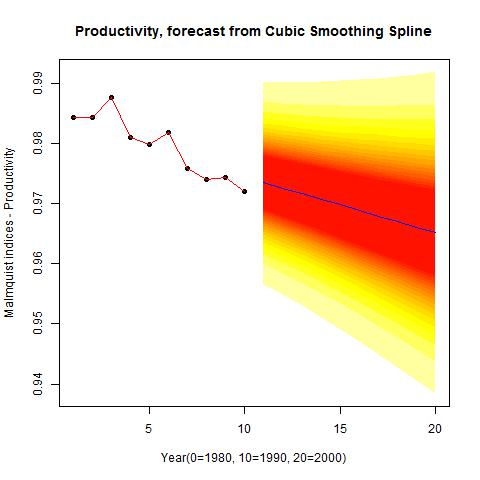#TECHNICAL EFFICIENCY fcast_tc <- splinef(tc.y,h=10, fan=T) plot(fcast_tc, main=”Technical Efficiency, forecast from Cubic Smoothing Spline”, ylab=”Malmquist indices – Technical Efficiency”, xlab=”Year(0=1980, 10=1990, 20=2000)”) # example – output graph to jpeg file jpeg(“…/Technical efficiency 1980_1990_Forecast Cubic_Smoothing_Spline10y2.jpg”) plot(fcast_tc, main=”Technical Efficiency, forecast from Cubic Smoothing Spline”, ylab=”Malmquist indices – Technical Efficiency”, xlab=”Year(0=1980, 10=1990, 20=2000)”) dev.off()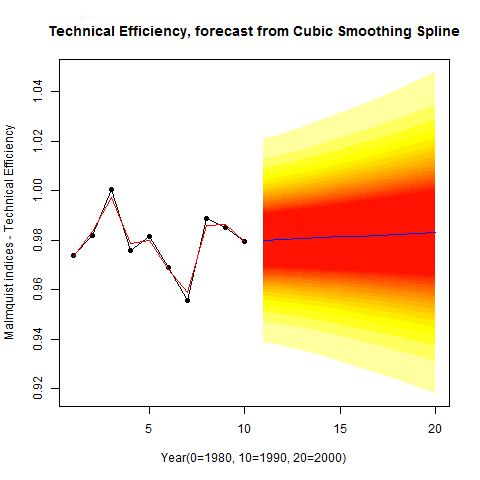#EFFICIENCY fcast_ec <- splinef(ec.y,h=10, fan=T) plot(fcast_ec, main=”Efficiency, forecast from Cubic Smoothing Spline”, ylab=”Malmquist indices – Efficiency”, xlab=”Year(0=1980, 10=1990, 20=2000)”) # example – output graph to jpeg file jpeg(“G:/data/home/2012/marko/blogi_rbloggerqvist/tekstiaihiot/40/Efficiency 1980_1990_Forecast Cubic_Smoothing_Spline10y2.jpg”) plot(fcast_ec, main=”Efficiency, forecast from Cubic Smoothing Spline”, ylab=”Malmquist indices – Efficiency”, xlab=”Year(0=1980, 10=1990, 20=2000)”) dev.off()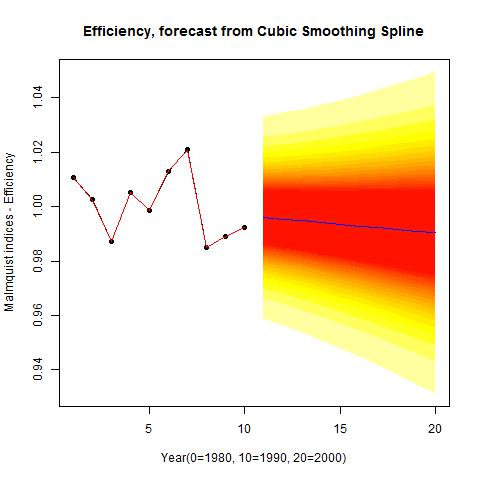summary(fcast_pc) summary(fcast_tc) summary(fcast_ec) ##################################################################### #2 #1991-2009 ##################################################################### #PRODUCTIVITY pc.y fcast_pc <- splinef(pc.y,h=10, fan=T) #NOTE confidence leve 50, 99 plot(fcast_pc, main=”Productivity, forecast from Cubic Smoothing Spline”, ylab=”Malmquist indices – Productivity”, xlab=”Year(0=1990, 20=2010)”) # example – output graph to jpeg file jpeg(“…/Productivity 1991_2009_Forecast Cubic_Smoothing_Spline10y2.jpg”) plot(fcast_pc, main=”Productivity, forecast from Cubic Smoothing Spline”, ylab=”Malmquist indices – Productivity”, xlab=”Year(0=1990, 20=2010)”) dev.off()#TECHNICAL EFFICIENCY fcast_tc <- splinef(tc.y,h=10, fan=T) plot(fcast_tc, main=”Technical Efficiency, forecast from Cubic Smoothing Spline”, ylab=”Malmquist indices – Technical Efficiency”, xlab=”Year(0=1990, 20=2010)”) # example – output graph to jpeg file jpeg(“G:/data/home/2012/marko/blogi_rbloggerqvist/tekstiaihiot/40/Technical efficiency 1991_2009_Forecast Cubic_Smoothing_Spline10y2.jpg”) plot(fcast_tc, main=”Technical Efficiency, forecast from Cubic Smoothing Spline”, ylab=”Malmquist indices – Technical Efficiency”, xlab=”Year(0=1990, 20=2010)”) dev.off()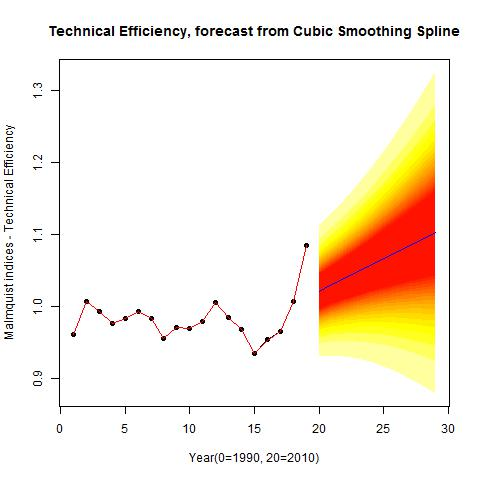#EFFICIENCY fcast_ec <- splinef(ec.y,h=10, fan=T) plot(fcast_ec, main=”Efficiency, forecast from Cubic Smoothing Spline”, ylab=”Malmquist indices – Efficiency”, xlab=”Year(0=1990, 20=2010)”) # example – output graph to jpeg file jpeg(“…/Efficiency 1991_2009_Forecast Cubic_Smoothing_Spline10y2.jpg”) plot(fcast_ec, main=”Efficiency, forecast from Cubic Smoothing Spline”, ylab=”Malmquist indices – Efficiency”, xlab=”Year(0=1990, 20=2010)”) dev.off()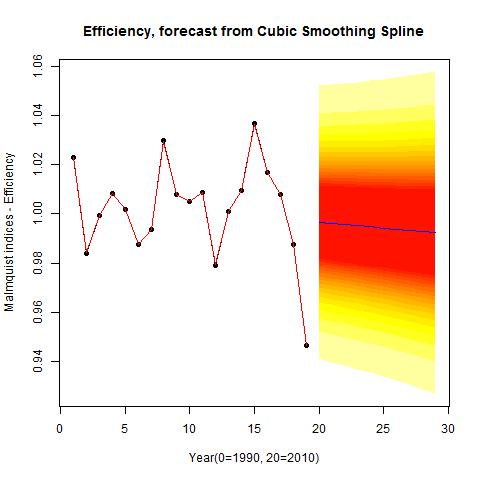summary(fcast_pc) summary(fcast_tc) summary(fcast_ec) ######################################################################3 #1991-2002 (capital included) # ##################################################################### #PRODUCTIVITY fcast_pc <- splinef(pc.y,h=10, fan=T) #NOTE confidence leve 50, 99 plot(fcast_pc, main=”Productivity, forecast from Cubic Smoothing Spline”, ylab=”Malmquist indices – Productivity”, xlab=”Year(1=1991, 10=2000, 20=2012)”) # example – output graph to jpeg file jpeg(“…/Productivity 1991_2002_Forecast Cubic_Smoothing_Spline10y2.jpg”) plot(fcast_pc, main=”Productivity, forecast from Cubic Smoothing Spline”, ylab=”Malmquist indices – Productivity”, xlab=”Year(1=1991, 10=2000, 20=2012)”) dev.off()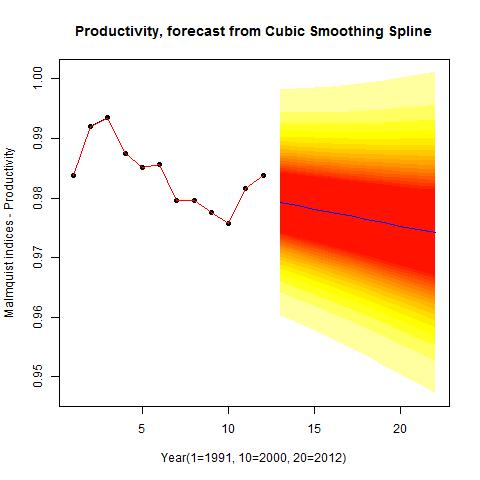#TECHNICAL EFFICIENCY fcast_tc <- splinef(tc.y,h=10, fan=T) plot(fcast_tc, main=”Technical Efficiency, forecast from Cubic Smoothing Spline”, ylab=”Malmquist indices – Technical Efficiency”, xlab=”Year(1=1991, 10=2000, 20=2012)”) # example – output graph to jpeg file jpeg(“…/Technical efficiency 1991_2002_Forecast Cubic_Smoothing_Spline10y2.jpg”) plot(fcast_tc, main=”Technical Efficiency, forecast from Cubic Smoothing Spline”, ylab=”Malmquist indices – Technical Efficiency”, xlab=”Year(1=1991, 10=2000, 20=2012)”) dev.off()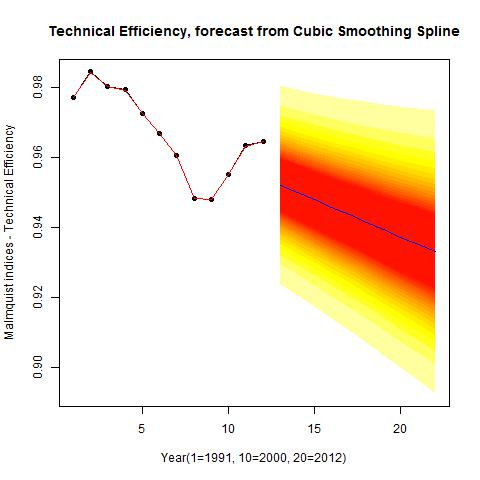#EFFICIENCY fcast_ec <- splinef(ec.y,h=10, fan=T) plot(fcast_ec, main=”Efficiency, forecast from Cubic Smoothing Spline”, ylab=”Malmquist indices – Efficiency”, xlab=”Year(1=1991, 10=2000, 20=2012)”) # example – output graph to jpeg file jpeg(“…/Efficiency 1991_2002_Forecast Cubic_Smoothing_Spline10y2.jpg”) plot(fcast_ec, main=”Efficiency, forecast from Cubic Smoothing Spline”, ylab=”Malmquist indices – Efficiency”, xlab=”Year(1=1991, 10=2000, 20=2012)”) dev.off()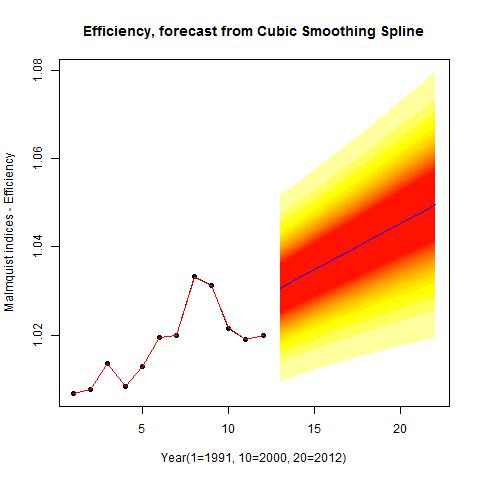summary(fcast_pc) summary(fcast_tc) summary(fcast_ec) ##################################################################### Ok, that’s it, Have fun with Linear Programming for the Malmquist Productivity Growth Index and its wide range of applications… Marko ## Presenting Mortality data with R and LateX # Mortality, fertility and migration What is the most informative way to present statistical dataset or actually time series data? Most convient way is to animate this data but how to do that with fastest and easiest way? Now I want to present one way to do that. Rob J Hyndman presented this data and animation technology in his blog on October 2010. Professor Hyndman presented French mortality data and how to utilize demography R-library.In this example I will introduce how to present mortality, fertility and migration data from Finland. As data source I will use data from here: http://www.mortality.org/ To get data you have to register as a user in The Human Mortality Database (HMD). Data source: Human Mortality Database. University of California, Berkeley (USA), and Max Planck Institute for Demographic Research (Germany). Available at www.mortality.org or www.humanmortality.de (data downloaded on 17.07.2012). Finnish death rate (mortality 1x1) data table name is Mx_1x1.txt and address is http://www.mortality.org/hmd/FIN/STATS/Mx_1x1.txt Of course you could also use hmd function to get data from HMD (included in demographic library) ie. norway <- hmd.mx("NOR", "user", "pass", "Norway") See more informaton about this from there. Any way I downloaded Finnish data (23 Mb) as bulk transfer after registering above mentioned MHD service. In this case I do the following things: STEP 1: fin_mortality <- read.demogdata("data/HMD/FIN/STATS/Mx_1x1.txt", "/data/HMD/FIN/STATS/Exposures_1x1.txt", type="mortality", label="Finland") STEP 2: #NEXT NOW WE WILL DO SEVERAL PDF FILES…… 🙂 #MALE nyears <- length(fin_mortality$year) for(i in 1:nyears)
{     pdf(paste("fimale",i,".pdf",sep=""),height=4,width=6.5)
x <- fin_mortality
if(i<nyears)         x$rate$male[,(i+1):nyears] <- NA
plot(x,series="male",ylim=c(-9.5,1.5),
main=paste("Finland: male mortality (",fin_mortality$year-1+i,")", sep="")) if(i>1) x$rate$male[,1:(i-1)] <- NA lines(x,series='male',lwd=2,col=1) dev.off() } #NEXT NOW WE WILL DO SEVERAL PDF FILES….AGAIN. 🙂 #FEMALE nyears <- length(fin_mortality$year) for(i in 1:nyears)
{     pdf(paste("fifemale",i,".pdf",sep=""),height=4,width=6.5)
x <- fin_mortality     if(i<nyears)
x$rate$female[,(i+1):nyears] <- NA
plot(x,series="female",ylim=c(-9.5,1.5),
main=paste("Finland: female mortality (",fin_mortality$year-1+i,")", sep="")) if(i>1) x$rate$female[,1:(i-1)] <- NA lines(x,series='female',lwd=2,col=1) dev.off() } #OK NOW LETS DO SOW TRICKS WITH LATEX STEP 3 Note! run this latex file at the same working directory as previous R step. In this example I use TexMakerX -program’s quick build –> and after that you will find animated pdf file from your working director. %Save this in the same working dir Note: replace fifemale text with fimale to produce male mortality animation. \documentclass{article} \usepackage{animate} \usepackage{graphicx} \begin{document} \begin{center} \centerline{\animategraphics[controls,buttonsize=0.3cm,width=12.5cm] {6}{"fifemale"}{1}{132}} \end{center} \end{document} STEP 4 Now You will find mortality pdf file animation male death rate and female death rate. Pdf file include “play” button, just press it and You will see mortality animation between year 1878 – 2009. How to make sense with line colours? Please read this article from Hyndman and Shang. http://robjhyndman.com/papers/Rainbow5.pdf “… the colors of the curves follow the order of a rainbow, with the oldest data in red and the most recent data in violet….” STEP 5 For Web publishing it is better to do gif animation: R and ImageMagick Note: install also these package if you encounter problems Microsoft Visual C++ 2010 Redistributable Package (x86) and Microsoft Visual C++ 2010 Redistributable Package (x64) package But before do that try to do following: Note for some reason (I do not know why) I have to set my path variables like this: C:\Program Files\ImageMagick; So, I create ImageMagick -folder and copy convert.exe from folder C:\Program Files (x86)\ImageMagick-6.7.8-Q16 …and everything go fine now. #In working director create a new folder and set the working directory to the new folder.  dir.create(“examples”) setwd(“examples”) library(animation) library(demography) #let’s check how many years we have nyears length(fin_mortality$year)
#now we will do as many frames as years we have

#MALE makeplot <- function(){ for(i in 1:nyears) {     x <- fin_mortality     if(i<nyears)         x$rate$male[,(i+1):nyears] <- NA     plot(x,series="male",ylim=c(-9.5,1.5),         main=paste("Finland: male mortality (",fin_mortality$year-1+i,")",sep="")) if(i>1) x$rate$male[,1:(i-1)] <- NA lines(x,series='male',lwd=2,col=1) } } oopt = ani.options(interval = 0, nmax = nyears) saveMovie(makeplot(),interval = 0.1, width = 580, height = 400) ani.options(oopt)  #FEMALE makeplot <- function(){ for(i in 1:nyears) { x <- fin_mortality if(i<nyears) x$rate$female[,(i+1):nyears] <- NA plot(x,series="female",ylim=c(-9.5,1.5), main=paste("Finland: female mortality (",fin_mortality$year-1+i,")",sep=""))     if(i>1)         x$rate$female[,1:(i-1)] <- NA     lines(x,series='female',lwd=2,col=1) } } oopt = ani.options(interval = 0, nmax = nyears) saveMovie(makeplot(),interval = 0.1, width = 580, height = 400) ani.options(oopt)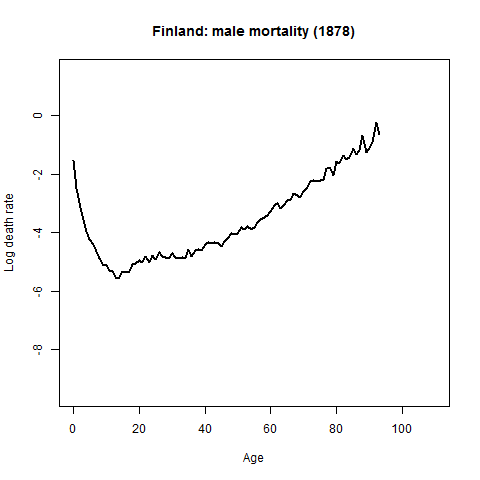# Visualize energy balance data

As energy engineer I have sometimes need to visualize energy balance data using Sankey diagram. In this example I will introduce how to do that with R.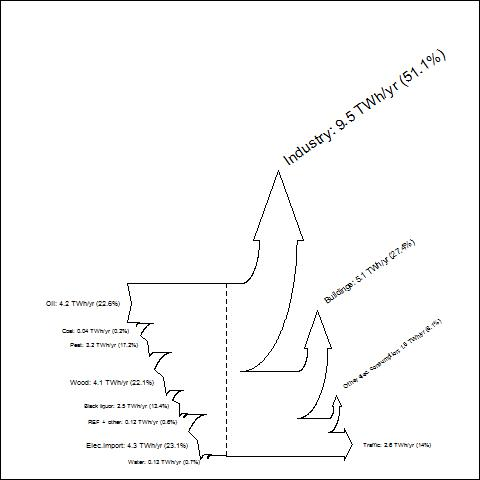As a data source I will use  Central Finlands energy balance 2010 data.

Source of data you will find from this address: Keski-Suomen energiatase 2010

Ok let’s start to rock with R

To produce Sankey with R we use Function SankeyR (you will find same source as I use in this example from here)

We run first SankeyR function. Copy following code into your RGui.

SankeyR <- function(inputs, losses, unit, labels, format="plot"){
########################
# SankeyR version 1.01 (updated August 10, 2010)
# is a function for creating Sankey Diagrams in R.
# See http://www.sankey-diagrams.com for excellent examples of Sankey Diagrams.
#
# OPTIONS:
# 'inputs' is a vector of input values
# 'losses' is a vector of loss values
# 'unit' is a string of the unit
# 'labels' is a vector of the labels for inputs and losses
# 'format' is the type of plotting:
#   The default is "plot," which produces a plot in the R graphics device.
#   Current alternate options include "pdf" and "bmp," which produce
#   those file types under the name "Sankey.xxx" in the current directory.
#
# Inputs do not need to equal losses.  Any difference will be displayed
# as a discrepancy in the height of the left and right sides of the diagram.
# This capability enables the developer to examine imbalances in flows.
# Percentages are a proportion of the inputs (so, the outputs might not equal 100%).
#
# EXAMPLE:
# Try using these values for the global carbon cycle, from Schlesinger (1997):
#  inputs = c(120,92)
#  losses = c(45,75,90,1,6)
#  unit = "GtC/yr"
#  labels = c("GPP","Ocean assimilation","Ra","Rh","Ocean loss","LULCC","Fossil fuel emissions")
#  SankeyR(inputs,losses,unit,labels)
#
# 8/10/10 - Added drawing for only one input.
#
# CREDITS:
# Created for R by Aaron BERDANIER
# send questions or comments to
# aaron.berdanier@gmail.com
#
# SankeyR is based strongly on drawSankey for Matlab,
# from James SPELLING, KTH-EGI-EKV (spelling@kth.se)
# http://leniwiki.epfl.ch/index.php/DrawSankey
#
# Licensees may copy, distribute, display, and perform the work and make
# derivative works based on it only for noncommercial purposes.
#
# Aaron would appreciate notification if you modify or improve this function.
########################
# Calculate fractional losses and inputs
frLosses = losses/sum(inputs)
frInputs = inputs/sum(inputs)
# First input and last output labels
inputLabel = paste(labels,": ",inputs," ",unit," (",round(100*frInputs,digits=1),"%)",sep="")
lossLabel = paste(labels[length(labels)],": ",losses[length(losses)]," ",unit,"
(",round(100*frLosses[length(losses)],digits=1),"%)",sep="")
########################
# Calculate position of plot axes (repeat of annotated code below; alternative: save values as vectors...)
limTop = frInputs; posTop = 0.4; maxy=0
limBot = 0; posBot = 0.1
if(length(inputs)>1){
for(j in 2:length(inputs)){
rI = max(0.07, abs(frInputs[j]/2))
rE = rI + abs(frInputs[j])
newPosB = posBot + rE*sin(pi/4) + 0.01
posBot = newPosB
arcEx = posBot - rE*sin(seq(0,pi/4,length.out=100))
arcEy = limBot - rE*(1-cos(seq(0,pi/4,length.out=100)))
arcIx = posBot - rI*sin(seq(0,pi/4,length.out=100))
arcIy = limBot - rE + rI*cos(seq(0,pi/4,length.out=100))
phiTip = pi/4 - 2*min(0.05, 0.8*abs(frInputs[j]))/(rI + rE)
xTip = posBot - (rE+rI)*sin(phiTip)/2
yTip = limBot - rE + (rE+rI)*cos(phiTip)/2
limBot = limBot - frInputs[j]
}
}else{}
posTop = posBot + 0.4
for(i in 1:(length(losses)-1)){
rI = max(0.07, abs(frLosses[i]/2))
rE = rI + abs(frLosses[i])
arcIx = posTop + rI*sin(seq(0,pi/2,length.out=100))
arcIy = limTop + rI*(1 - cos(seq(0,pi/2,length.out=100)))
arcEx = posTop + rE*sin(seq(0,pi/2,length.out=100));
arcEy = (limTop + rI) - rE*cos(seq(0,pi/2,length.out=100))
arEdge = max(0.015, rI/3)
arTop  = max(0.04, 0.8*frLosses[i])
arX = posTop + rI + c(0,-arEdge,frLosses[i]/2,frLosses[i]+arEdge,frLosses[i])
arY = limTop + rI + c(0,0,arTop,0,0)
if(max(arY)>maxy){maxy = max(arY)}else{maxy=maxy}
limTop = limTop - frLosses[i]
newPos = posTop + rE + 0.01
posTop = newPos
}
newPos = max(posTop, posBot) + max(0.05*limTop,0.05)
newPos = newPos + 0.8*(limTop-limBot)
maxx = newPos
miny = (limTop-frLosses[length(frLosses)])-max(0.015,abs(frLosses[length(frLosses)]/4))
maxy = maxy*2
minx = 0
########################
# Graphics type?
if(format!="plot"){
# Call graphics device
plottype = switch(format,
"pdf" = pdf("Sankey.pdf", width=11, height=min(8.5,11*(maxy-miny)/((maxx+3)-minx))),
"bmp" = bmp("Sankey.bmp", width=800*((maxx+3)-minx)/(maxy-miny), height=800, unit="px",res=144)
)
}
# Create plotting window
par(mar=c(0,0,0,0),oma=c(0,0,0,0))
plot(0,0,type="n",xlim=c(-1.5,maxx+1.5),ylim=c(miny,maxy),xaxt="n",yaxt="n")
w = 1 # line width
# Calculate fractional losses and inputs
frLosses = losses/sum(inputs)
frInputs = inputs/sum(inputs)
# Draw back edge of first input arrow
lines(c(0.1,0,0.05,0,0.4), c(0,0,frInputs/2,frInputs,frInputs),lwd=w)
# First input label
inputLabel = paste(labels,": ",inputs," ",unit," (",round(100*frInputs,digits=1),"%)",sep="")
fontsize = max(0.5,frInputs*2.5)
text(0, frInputs/2, inputLabel, cex=fontsize, pos=2) # try pos=4
# Set initial position for the top of the arrows
limTop = frInputs; posTop = 0.4; maxy=0
# set initial position for the bottom of the arrows
limBot = 0; posBot = 0.1
###
# DRAW ARROWS FOR ADDITIONAL INPUTS
if(length(inputs)>1){
for(j in 2:length(inputs)){
# determine inner and outer arrow radii
rI = max(0.07, abs(frInputs[j]/2))
rE = rI + abs(frInputs[j])
# push separation point forwards
newPosB = posBot + rE*sin(pi/4) + 0.01
lines(c(posBot,newPosB), c(limBot,limBot), lwd=w)
posBot = newPosB
# determine points on the external arc
arcEx = posBot - rE*sin(seq(0,pi/4,length.out=100))
arcEy = limBot - rE*(1-cos(seq(0,pi/4,length.out=100)))
# determine points on the internal arc
arcIx = posBot - rI*sin(seq(0,pi/4,length.out=100))
arcIy = limBot - rE + rI*cos(seq(0,pi/4,length.out=100))
# draw internal and external arcs
lines(arcIx, arcIy, lwd=w)
lines(arcEx, arcEy, lwd=w)
# determine arrow point tip
phiTip = pi/4 - 2*min(0.05, 0.8*abs(frInputs[j]))/(rI + rE)
xTip = posBot - (rE+rI)*sin(phiTip)/2
yTip = limBot - rE + (rE+rI)*cos(phiTip)/2
# draw back edge of additional input arrows
lines(c(min(arcEx),xTip,min(arcIx)), c(min(arcEy),yTip,min(arcIy)), lwd=w)
# Draw label
phiText = pi/2-2*min(0.05,0.8*abs(frInputs[j]))/(rI+rE)
xText = posBot-(rE+rI)*sin(phiText)/3
yText = limBot-rE/1.5+(rE+rI)*cos(phiText)/2
fullLabel = paste(labels[j],": ",inputs[j]," ",unit," (",round(100*frInputs[j],digits=1),"%)",sep="")
fontsize = max(0.5,frInputs[j]*2.5)
text(xText, yText, fullLabel, cex=fontsize, pos=2)
# save new bottom end of arrow
limBot = limBot - frInputs[j]
}
posTop = posBot + 0.4
lines(c(0.4,posTop), c(frInputs,frInputs), lwd=w)
lines(c(posBot,posBot+(posTop-posBot)/2), c(limBot,limBot),lwd=w)
posMid=posBot+(posTop-posBot)/2
}else{
lines(c(posBot,posBot+(posTop-posBot)/2), c(limBot,limBot),lwd=w)
posMid=posBot+(posTop-posBot)/2
}
###
# DRAW ARROWS OF LOSSES
for(i in 1:(length(losses)-1)){
# Determine inner and outer arrow radii
rI = max(0.07, abs(frLosses[i]/2))
rE = rI + abs(frLosses[i])
# Determine points on the internal arc
arcIx = posTop + rI*sin(seq(0,pi/2,length.out=100))
arcIy = limTop + rI*(1 - cos(seq(0,pi/2,length.out=100)))
# Determine points on the internal arc
arcEx = posTop + rE*sin(seq(0,pi/2,length.out=100));
arcEy = (limTop + rI) - rE*cos(seq(0,pi/2,length.out=100))
# Draw internal and external arcs
lines(arcIx, arcIy, lwd=w)
lines(arcEx, arcEy, lwd=w)
# Determine arrow tip dimensions
arEdge = max(0.015, rI/3)
arTop  = max(0.04, 0.8*frLosses[i])
# Determine points on arrow tip
arX = posTop + rI + c(0,-arEdge,frLosses[i]/2,frLosses[i]+arEdge,frLosses[i])
arY = limTop + rI + c(0,0,arTop,0,0)
if(max(arY)>maxy){maxy = max(arY)}else{maxy=maxy}
# Draw tip of losses arrow
lines(arX, arY, lwd=w)
# Draw label
txtX = posTop + rI + frLosses[i]/2
txtY = limTop + rI + arTop + 0.05
fullLabel = paste(labels[i+length(inputs)],": ",losses[i]," ",unit,"
(",round(100*frLosses[i],digits=1),"%)",sep="")
fontsize = max(0.5,frLosses[i]*2.5)
text(txtX, txtY, fullLabel, cex=fontsize, pos=4, srt=35)
# Save new position of arrow top
limTop = limTop - frLosses[i]
# Advance to new separation point
newPos = posTop + rE + 0.01
# Draw top line to new separation point
lines(c(posTop,newPos), c(limTop,limTop), lwd=w)
posTop = newPos
# SEPARATION LINES - not implemented yet
}
###
# Push the arrow forwards a little after all side-arrows drawn
newPos = max(posTop, posBot) + max(0.05*limTop,0.05)
# Draw lines to this new position
lines(c(posTop,newPos), c(limTop,limTop),lwd=w)
lines(c(posMid,newPos), c(limTop-frLosses[length(frLosses)],limTop-frLosses[length(frLosses)]),lwd=w)
# Draw final arrowhead for the output
lines(c(newPos,newPos,newPos+max(0.04,0.8*(frLosses[length(frLosses)])),newPos,newPos),
c(limTop,limTop+max(0.015,abs(frLosses[length
(frLosses)]/6)),limTop-frLosses[length(frLosses)]/2,(limTop-frLosses[length(frLosses)])-max(0.015,
abs(frLosses[length(frLosses)]/6)),(limTop-frLosses
[length(frLosses)])),  lwd=w)
# Save final tip position
newPos = newPos + 0.8*(frLosses[length(frLosses)])
# Last loss label
lossLabel = paste(labels[length(labels)],": ",losses[length(losses)]," ",unit,"
(",round(100*frLosses[length(losses)],digits=1),"%)",sep="")
fontsize = max(0.5,frLosses[length(losses)]*2.5)
text(newPos+0.05, limTop-frLosses[length(frLosses)]/2, lossLabel, cex=fontsize, pos=4) # try pos=4
# Draw mid-line
if(limBot<(limTop-frLosses[length(frLosses)])){
lines(c(posMid,posMid), c(frInputs,limBot),lty=2)
}else{
lines(c(posMid,posMid), c(frInputs,limTop-frLosses[length(frLosses)]),lty=2)
}
if(format!="plot"){
# Close graphics device
dev.off()
}}

After that “Gui-run” we use Central Finlands energy balance data from year 2010…

#Central Finlands energy balance 2010 (Keski-Suomen energiatase 2010 Source: http://www.kesto.fi)
#
# inputs
#Oil 4.2 TWh
#Coal 0,04
#Peat 3.2
#Wood fuel 4.1
#Black ? 2.5
#REF + other 0.12
#Water 0.13
#Elec.import 4.3
# lossess
#Industry 9.5
#--> Elec 4,65
#--> process heat 4,85
#Buildings 5.1
#--> district heating 2.55
#--> wood 0.663
#--> oil 0.969
#--> elec 0,867
#Other elec consumption 1.5
#Traffic 2.6
#--> Bensin  1.196
#--> Diesel 1.404
#total 18.6 TWh
inputs = c(4.2,0.04,3.2,4.1,2.5,0.12,4.3,0.13)
losses = c(9.5,5.1,1.5,2.6)
unit = "TWh/yr"
labels = c("Oil","Coal","Peat","Wood","Black liquor","REF + other","Elec.import","Water",
"Industry","Buildings", "Other elec. consumption", "Traffic")
SankeyR(inputs,losses,unit,labels)
#saving into file jpg. note: picture quality not so good
jpeg(".../energybalance.jpg")
SankeyR(inputs,losses,unit,labels)
dev.off()
#saving into file note: picture quality not so good
png(".../energybalance.png")
SankeyR(inputs,losses,unit,labels)
dev.off()
#saving into file note: note: picture quality not so good
bmp(".../energybalance.bmp")
SankeyR(inputs,losses,unit,labels)
dev.off()
#saving into file note: picture quality excellent
pdf(".../energybalance.pdf")
SankeyR(inputs,losses,unit,labels)
dev.off()

Result is ok, but just now I have no idea how to produce “sub-branches” of losses. Anyway very nice routine to produce Sankey….And here you will find Sankey diagram in pdf format energybalance.

Have fun,
Marko

# R and Eurostat bulk data

In this Exercise I am testing Eurostat bulk data source and plot these data into Heatmap. Let’s try with this data:
“Harmonised unemployment rates (%) – monthly data (ei_lmhr_m)”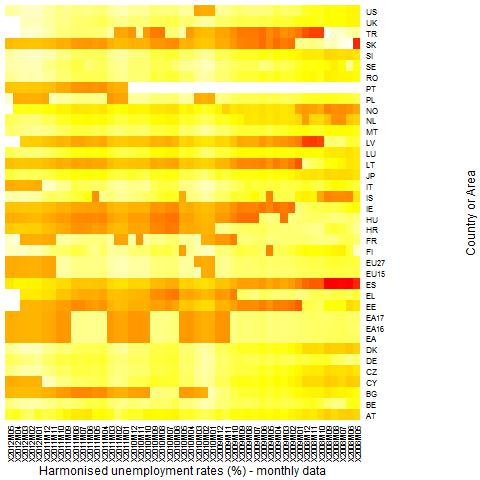#You will also find Eurostat Data source from here:

 #I will automate this data downloading and extracting, but just now this is semiautomatic # create download directory and set it .exdir = 'c:/data/tmp2' # put there your own data folder dir.create(.exdir) .file = file.path(.exdir, 'ei_lmhr_m.tsv.gz') # change this  # download file url = 'http://epp.eurostat.ec.europa.eu/NavTree_prod/everybody/BulkDownloadListing?sort=1&downfile=data  %2Fei_lmhr_m.tsv.gz' download.file(url, .file)  # untar it (Note: I do not know why I got error message: Error in getOct(block, 100, 8) : invalid octal digit) untar(.file, compressed = 'gzip', exdir = path.expand(.exdir))  # Argh...something going wrong with this step, so I have to manipulate just downloaded data. First I remove comma  # from very first variables and etc... I always use Notetab light as a Text editor in this kind of task.  # Reading file into R. Please refer here your own data folder... input <- read.table("c:/data/tmp2/ei_lmhr_m.tsv", header=TRUE, sep="\t", na.strings=":", dec=".", strip.white=TRUE)  #just checking head(input)   # LM-UN-T-TOT = Unemployment rate according to ILO definition - Total rate # NSA = not seasonally adjusted input<- input[which(input$indic=="LM-UN-T-TOT"),] input<- input[which(input$s_adj=="NSA"),]  #giving appropriate names in to the heatmap (without this manouver there will be only row id) row.names(input) <- input$geo.time  #just checking head(input)  #Column selection. We will get data between time period 05/2008 - 05/2012  input2 <- input[,5:53]  # data frame must change into data matrix to produce heatmap. input_matrix <- data.matrix(input2)  #heatmap is almost here input_heatmap <- heatmap(input_matrix, Rowv=NA, Colv=NA, col = heat.colors(256), scale="column", margins=c  (5,10), xlab = "Harmonised unemployment rates (%) - monthly data", ylab= "Country or Area")   #saving heatmap into folder jpeg("G:/data/home/2012/marko/blogi_rbloggerqvist/data/eurostat/Harmonised unemployment rates percent  monthly data.jpg") input_heatmap <- heatmap(input_matrix, Rowv=NA, Colv=NA, col = heat.colors(256), scale="column", margins=c  (5,10), xlab = "Harmonised unemployment rates (%) - monthly data", ylab= "Country or Area") dev.off()  Have fun, Marko ## R and population maps in Finland 31.5.2012 This example source is almost same like this. I used at first time sorvi-package. In this example I modified little bit original and try to compare MML and GADM shape – files. As we know there is some need to updates into GADM shapefiles (as you will see). Datafiles source date is 31.05.2012 from Väestörekisterikeskus. Thanks to Louhos -people and their invaluable work within open -data project. # library sorvi You will find there library(sorvi) library(rgeos) library(rgdal) if (!gpclibPermit()) { gpclibPermit() } # Finland map and municipility data at gadm-format gadm <- GetGADM(“FIN_adm”, “Kunta”) ##now we use also MML as data source (shape) because of compare shape files # (C) MML 2011 data(MML) sp <- MML[[“1_milj_Shape_etrs_shape”]][[“kunta1_p”]] # vaestorekisteri population data from data source vrk.fi 31.05.2012 vrek <- GetPopulationRegister(“http://vrk.fi/default.aspx?docid=6706&site=3&id=0”) # Attach vrk data into map object and # set population as zero where na gadm$asukkaita <- log10(rowSums(vrek[gadm$Kunta, c(“Miehet”, “Naiset”)])) gadm$asukkaita[is.na(gadm$asukkaita)] <- 0 sp$asukkaita <- log10(rowSums(vrek[sp$Kunta, c(“Miehet”, “Naiset”)])) sp$asukkaita[is.na(sp$asukkaita)] <- 0 # male and female share gadm$miehet.osuus <- vrek[gadm$Kunta, “Miehet”]/vrek[gadm$Kunta, “Yhteensa”]
gadm$naiset.osuus <- vrek[gadm$Kunta, “Naiset”]/vrek[gadm$Kunta, “Yhteensa”] gadm_summary_male <- summary(vrek[gadm$Kunta, “Miehet”]/vrek[gadm$Kunta, “Yhteensa”]) gadm_summary_female <- summary(vrek[gadm$Kunta, “Naiset”]/vrek[gadm$Kunta, “Yhteensa”]) sp$miehet.osuus <- vrek[sp$Kunta, “Miehet”]/vrek[sp$Kunta, “Yhteensa”]
sp$naiset.osuus <- vrek[sp$Kunta, “Naiset”]/vrek[sp$Kunta, “Yhteensa”] sp_summary_male <- summary(vrek[sp$Kunta, “Miehet”]/vrek[sp$Kunta, “Yhteensa”]) sp_summary_female <- summary(vrek[sp$Kunta, “Naiset”]/vrek[sp$Kunta, “Yhteensa”]) #some summary stat gadm_summary_male gadm_summary_female sp_summary_male sp_summary_female hist_gadm_male <- hist(gadm$miehet.osuus)
hist_gadm_female <- hist(gadm$naiset.osuus) hist_sp_male <- hist(sp$miehet.osuus)
hist_sp_female <- hist(sp$naiset.osuus) plot(density(gadm$miehet.osuus,na.rm=TRUE))
plot(density(gadm$naiset.osuus,na.rm=TRUE)) plot(density(sp$miehet.osuus,na.rm=TRUE))
plot(density(sp$naiset.osuus,na.rm=TRUE)) #saving hist figure into file …\r_maps jpeg(“…/hist.jpg”) layout(matrix(1:4,2,2)) hist_gadm_male <- hist(gadm$miehet.osuus)
hist_gadm_female <- hist(gadm$naiset.osuus) hist_sp_male <- hist(sp$miehet.osuus)
hist_sp_female <- hist(sp$naiset.osuus) dev.off()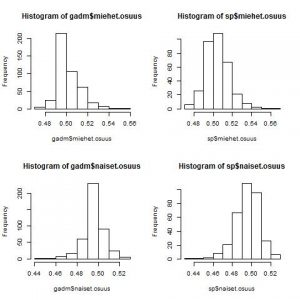#saving density figre into file …\r_maps (replace … as your fav. folder) jpeg(“…/density.jpg”) layout(matrix(1:4,2,2)) plot(density(gadm$miehet.osuus,na.rm=TRUE))
plot(density(gadm$naiset.osuus,na.rm=TRUE)) plot(density(sp$miehet.osuus,na.rm=TRUE))
plot(density(sp$naiset.osuus,na.rm=TRUE)) dev.off()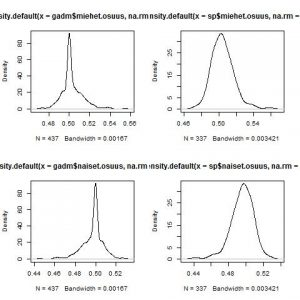# set share 50% male/female # where na gadm$miehet.osuus[is.na(gadm$miehet.osuus)] <- 0.5 gadm$naiset.osuus[is.na(gadm$naiset.osuus)] <- 0.5 #same with MML sp$miehet.osuus[is.na(sp$miehet.osuus)] <- 0.5 sp$naiset.osuus[is.na(sp$naiset.osuus)] <- 0.5 # border of interval GADM varname1 <- “naiset.osuus” interval1 <- max(abs(gadm[[varname1]] – 0.5)) at1 <- seq(0.5 – interval1, 0.5 + interval1, length = 100) #border of interval MML varname2 <- “naiset.osuus” interval2 <- max(abs(sp[[varname2]] – 0.5)) at2 <- seq(0.5 – interval2, 0.5 + interval2, length = 100) #PLOTTING INTO FILE jpeg(“j:/todo/r_maps/gadm_280612.jpg”) q1 <- PlotShape(gadm, varname1, type = “twoway”, at = at1, main = “Share of women (red) and men (blue) 31.05.2012 n=437 (GADM)”) dev.off() jpeg(“j:/todo/r_maps/mml_280612.jpg”) q2 <- PlotShape(sp, varname2, type = “twoway”, at = at2, main = “Share of women (red) and men (blue) 31.05.2012 n=337 (MML)”) dev.off() #Which one is best?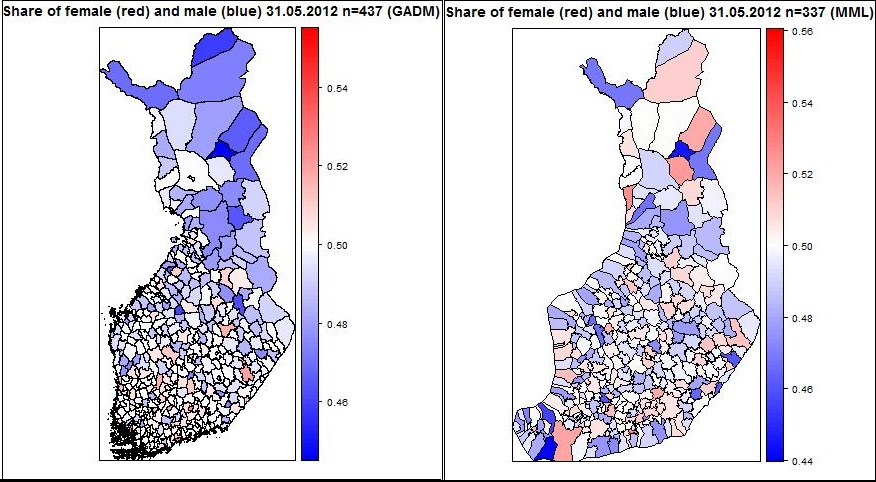GADM projection is not like “normal” and MML coastline is little bit odd, but I am patiently looking forward to improvement into this issue…. ME/290612 ## R and VIX index # Using R as visualizing Volatility Index VIX On September 22, 2003, the CBOE began disseminating price level information using revised methodology for the CBOE Volatility Index, VIX. A spreadsheet with more than 13 years of price history data using this new methodology is now available. ## VIX? In 1993, the Chicago Board Options Exchange® (CBOE®) introduced the CBOE Volatility Index®, VIX®, and it quickly became the benchmark for stock market volatility. It is widely followed and has been cited in hundreds of news articles in the Wall Street Journal, Barron’s and other leading financial publications. Since volatility often signifies financial turmoil, VIX is often referred to as the “investor fear gauge”. Source: http://www.cboe.com/micro/vix/faq.aspx#1 So, I found this example from there. I change it little bit. In this exampele it use whole time series you will found there. Now let’s look at closer this amazing approach…  #required library require(quantmod) require(ggplot2) require(reshape2) require(plyr) require(scales)  # download data source input <- read.table("http://www.cboe.com/publish/ScheduledTask/MktData/datahouse/vixcurrent.csv", header=TRUE, sep=",", na.strings="NA", dec=".", strip.white=TRUE)  # creating dataframe dat<-data.frame(date=index(VIX),VIX) #look what we get head(dat)  #date conversation input$date <- as.Date(input$Date, "%m/%d/%Y")  ## below some example do to that ## read in date/time info in format 'm/d/y h:m:s' ## dates <- c("02/27/92", "02/27/92", "01/14/92", "02/28/92", "02/01/92") ## times <- c("23:03:20", "22:29:56", "01:03:30", "18:21:03", "16:56:26") ## x <- paste(dates) ## y <- strptime(x, "%m/%d/%y")  # extract year input$year<-as.numeric(as.POSIXlt(input$date)$year+1900)  # and  the month too OK input$month<-as.numeric(as.POSIXlt(input$date)$mon+1)  # monts into right order and giving factor name input$monthf<-factor(input$month,levels=as.character(1:12),labels=c("Jan","Feb","Mar","Apr","May","Jun","Jul","Aug","Sep","Oct","Nov","Dec"),ordered=TRUE)  # week days input$weekday = as.POSIXlt(input$date)$wday  # week day ordering and gibing factor name (weekdays) input$weekdayf<-factor(input$weekday,levels=rev(1:7),labels=rev(c("Mon","Tue","Wed","Thu","Fri","Sat","Sun")),ordered=TRUE)  # yearmonth input$yearmonth<-as.yearmon(input$date) input$yearmonthf<-factor(input$yearmonth)  # week of year by rows input$week <- as.numeric(format(input$date,"%W"))  # making week to start at number 1 input<-ddply(input,.(yearmonthf),transform,monthweek=1+week-min(week))  # Ok, Now we are ready to plot  P<- ggplot(input, aes(monthweek, weekdayf, fill = VIX.Close)) + geom_tile(colour = "white") + facet_grid(year~monthf) + scale_fill_gradient(low="yellow", high="red") + opts(title = "Heatmap - view of future expected stock market volatility (risk)") + xlab("Week of Month") + ylab("")  #show the plot P  # save the picture # example - output graph to jpeg file jpeg("heatmaps.jpg") P dev.off()  ...and output will look like this: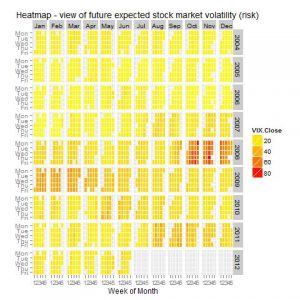That’s it,

Cheers, Marko

# Earth quakes of the last 30 days

These example come from here. I follow instruction how to plot earthquage data into world map between 24.5. – 23.6.2012. My first test is not succesfull, so I make my copy paste tricks. Final result show now “right” magnitude.## Plot world wide earth quakes of the last 30 days with magnitude >= 4.0 library(googleVis) library(XML) ## Get earthquake data of the last 30 days ## That is ok, put for some reason I do not get right magnitude into picture. eq=readHTMLTable(readLines("http://www.iris.edu/seismon/last30.html")) eq <- eq[] ## extract the eq table summary(eq) #something wrong with this  last30 file #I have to copy and paste web-page data into Notepad and saved this into file earthquake.txt #eq <- read.table("j:/todo/R_geodata/earthquake.txt", header=TRUE, sep="\t", na.strings="NA", dec=".", strip.white=TRUE) #WEB-server datafile note I change MAG into magni eq <- read.table("http://ekqvist.goeuropeinfo.com/rbloggerqvist/data/earthq/earthquake.txt", header=TRUE, sep="\t", na.strings="NA", dec=".", strip.white=TRUE)

that’s it…